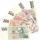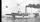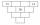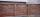# Examples for 9th grade - page 71

1. CandyThe price for 1kg of more expensive candy is 125 CZK. The price for 1kg of cheaper candy is 100 CZK. We make two different blends of candy. And now. The second blend contains 2kg of more expensive candy and several kg of cheaper candy. The price per 1 kg o
2. Saving per centsThe first day I save 1 cent and every next day cent more. How many I saved per year (365 days)?
3. Centre of massThe vertices of triangle ABC are from the line p distances 3 cm, 4 cm and 8 cm. Calculate distance from the center of gravity of the triangle to line p.
4. ResistanceDetermine the resistance of the bulb with current 200 mA and is in regular lamp (230V).
5. Diameters of circlesHow many percent of the area of a larger circle is a smaller circle if the smaller circle has a diameter 120 mm and a larger one has a diameter 300 mm?
6. Cruise linerCruise liner runs along the river between points A and B. The journey downstream takes 40 minutes 1 hour upriver. The flow rate of the river is 3 km/h. What is the speed of cruise liner?
7. Family parcelsIn father will he divided the land so that the older son had three bigger part than younger son. Later elder son gave 2.5 ha field to younger and they had both the same. Determine the area of family parcel.
8. Diagonals of diamondFind the area and circumference of the diamond ABCD with 15m and 11m diagonals.
9. Pyramid Z8–I–6Each brick of pyramid contains one number. Whenever possible, the number in each brick is lowest common multiple of two numbers of bricks lying directly above it. That number may be in the lowest brick? Determine all possibilities.
10. Cost reductionTwo MP3 players whose price was equal to originally have been discounted the first by 20%, the second by 35%. After the price reduction was the difference in their prices 750, - CZK. What was the original price of each of the two players?
11. Isosceles trapezoidCalculate the circumference and the contents of the isosceles trapezoid if you know the size of the bases is 8 and 12 cm and the size of the arms is 5 cm.
12. Two numbersThe sum of the two numbers is 1. Find both numbers if you know that half of the first is equal to one seventh of the second number.
13. Cuboid edges in ratioCuboid edges lengths are in ratio 2:4:6. Calculate their lengths if you know that the cuboid volume is 24576 cm3.
14. Is right triangleDecide if the triangle XYZ is rectangular: x = 4 m, y = 6 m, z = 4 m
15. Brick wallGarden 70 m long and 48 m wide should surround with wall 2.1 meters high and 30 cm thick. Wall will be built on the garden ground. How many will we need bricks if to 1 m³ is required approximately 300 bricks?
16. Rabbits 3Viju has 40 chickens and rabbits. If in all there are 90 legs. How many rabbits are there with Viju?
17. Two numbersWe have two numbers. Their sum is 140. One-fifth of the first number is equal to half the second number. Determine those unknown numbers.
18. Land - isosceles trapezoidCalculate the content and perimeter of the building plot in the form of an isosceles trapezoid with bases 120m, 95m and height 50m.
19. Timber logThe ship goes from point x to y. Downstream it takes 4 hours, upstream 6 hours. How long does it take from a point x to y a log?
20. TreeBetween points A and B is 50m. From A we see a tree at an angle 18°. From point B we see the tree in three times bigger angle. How tall is a tree?

Do you have an interesting mathematical word problem that you can't solve it? Enter it, and we can try to solve it.

To this e-mail address, we will reply solution; solved examples are also published here. Please enter the e-mail correctly and check whether you don't have a full mailbox.

Please do not submit problems from current active competitions such as Mathematical Olympiad, correspondence seminars etc...